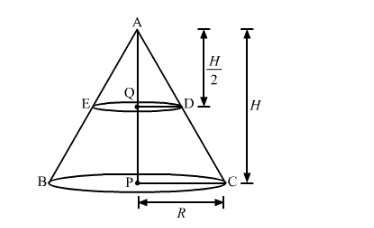# If a cone of radius 10 cm is divided into two`
Question:

If a cone of radius 10 cm is divided into two parts by drawing a plane through the mid-point of its axis, parallel to its base. Compare the volumes of the two parts.

Solution:Consider a cone of radius R and height H.

Let a cone of height $\frac{\mathrm{H}}{2}$ is cutout from this cone whose base is parallel to the original cone. Now is

$\triangle A Q D$ and $\triangle A P C, Q D \| P C$

$\Delta A Q D-\Delta A P C$

$\frac{Q D}{P C}=\frac{A Q}{A P}$

$\frac{Q D}{2}=\frac{H}{\frac{2}{H}}$

$Q D=\frac{R}{2}$

Volume of cone $\mathrm{ABC}=\frac{1}{3} \pi R^{2} \mathrm{H}$

$=\frac{1}{3} \pi\left(\frac{R}{2}\right)^{2}\left(\frac{H}{2}\right)$

$=\frac{1}{8}\left[\frac{1}{3} \pi R^{2} H\right]$

$=\frac{1}{8}$ volume of cone $\mathrm{ABC}$

Now volume of remaining frustum of cons EDCB

= volume of cone ABC − volume of cone AED

$=\frac{7}{8}($ volume of cone $A B C)$

Comparing the volume of cone AED and frustum EDBC we get, the ratio 1 : 7.